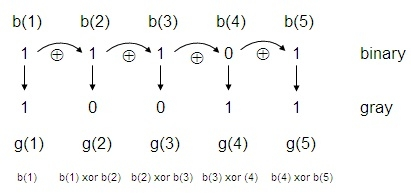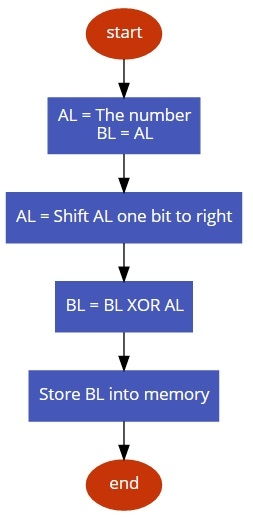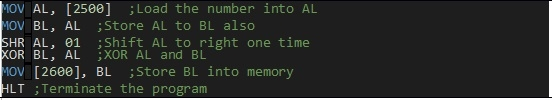# 8086 program to convert binary to Grey code

In this program we will see how to find the gray code from a binary number.

## Problem Statement

Write 8086 Assembly language program to find the equivalent gray code from a binary number. The number is stored at location 2500 and store the result at 2600.

## Discussion

To convert binary to gray code, we have to shift the number one bit to the right, then XOR with the previous number. Thus the gray code will be generated.For a number 2C (0010 1100) the gray code will be 3A (0011 1010)

Data

2500
2C

## Flow Diagram## Program